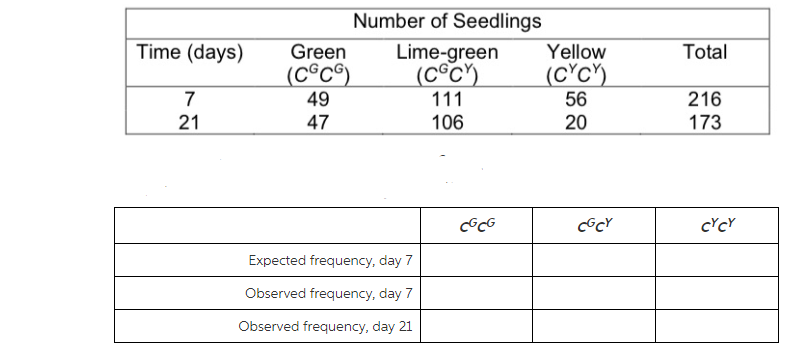# Problem: Using the day 7 data, what is the frequency of the CGallele (p)?Using the day 7 data, what is the frequency of the CYallele (q)?

###### Problem Details

Using the day 7 data, what is the frequency of the CGallele (p)?

Using the day 7 data, what is the frequency of the CYallele (q)?What scientific concept do you need to know in order to solve this problem?

Our tutors have indicated that to solve this problem you will need to apply the Hardy-Weinberg Model concept. You can view video lessons to learn Hardy-Weinberg Model. Or if you need more Hardy-Weinberg Model practice, you can also practice Hardy-Weinberg Model practice problems.

What is the difficulty of this problem?

Our tutors rated the difficulty ofUsing the day 7 data, what is the frequency of the CGallele ...as high difficulty.

How long does this problem take to solve?

Our expert Biology tutor, Kaitlyn took 18 minutes and 13 seconds to solve this problem. You can follow their steps in the video explanation above.

What professor is this problem relevant for?

Based on our data, we think this problem is relevant for Professor Rafferty's class at BMCC CUNY.